Question

A. Calculate the pH of a solution containing 0.2M lactic acid (pKa = 3.86)

B. Calculate the pH of a solution of 1x10^-9 M HCl (strong acid) in distilled water. Show your work.

The answer is given as follows:-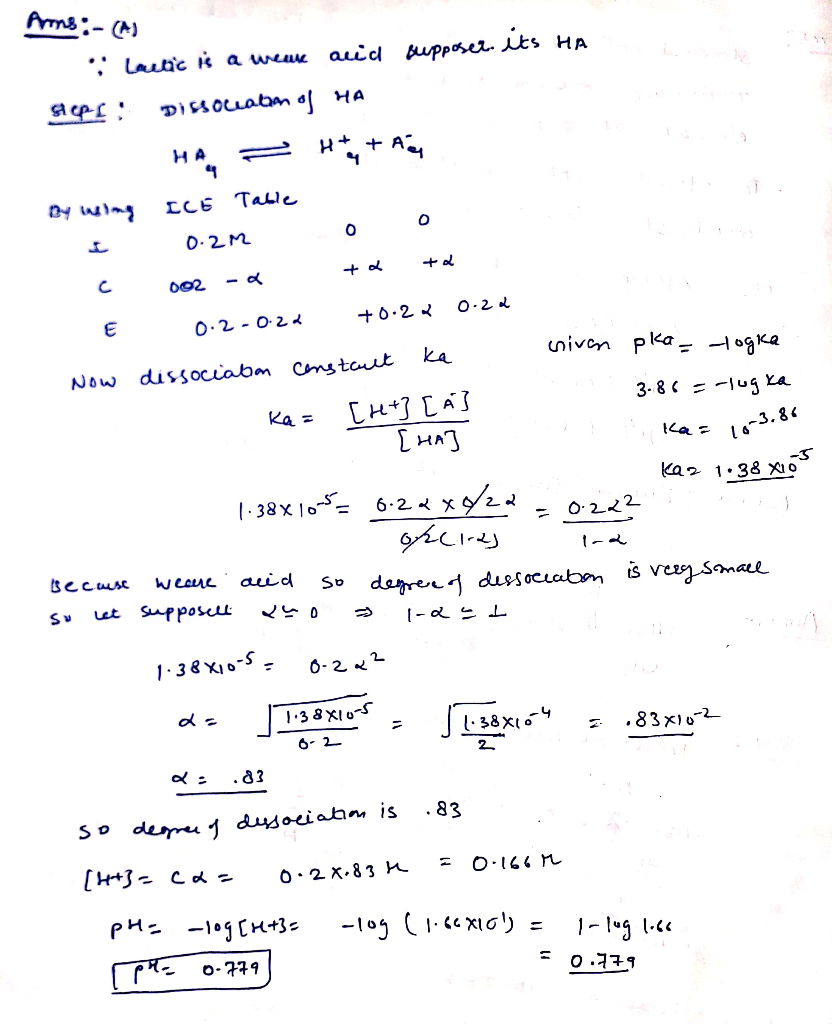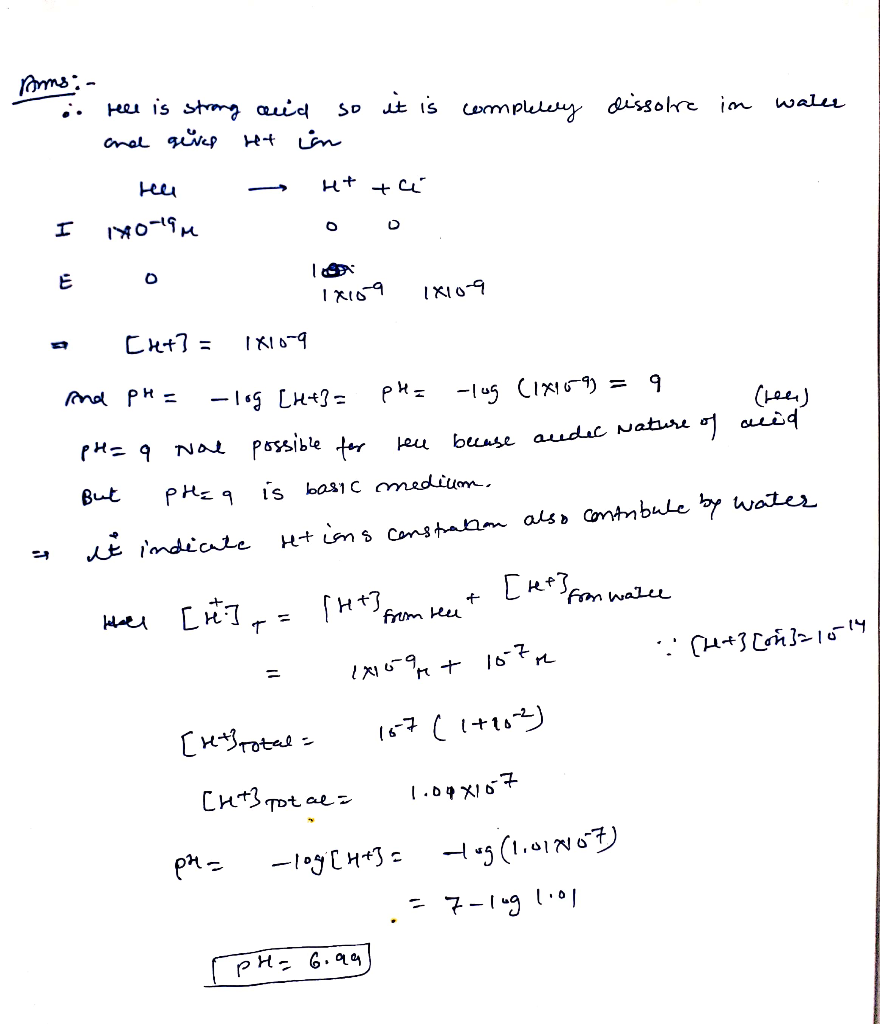#### Earn Coins

Coins can be redeemed for fabulous gifts.

Similar Homework Help Questions
• ### Given stocks of 1 M Lactic Acid (pKa 3.86) at pH 3.5, 2 M naOH, 2...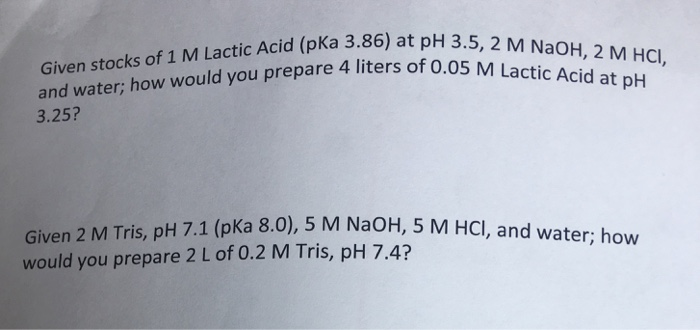Given stocks of 1 M Lactic Acid (pKa 3.86) at pH 3.5, 2 M naOH, 2 M HCl and water: how would you prepare 4 liters of 0.05 M Lactic Acid at pH 3.25? Give 2 M Tris, pH 7.1 (pKa 8.0), 5 M NaOH, 5 M HCl, and water: how would you prepare 2 L of 0.2 M Tris, pH 7.4?

• ### A buffer contains 0.010 mol of lactic acid (pKa = 3.86) and 0.050 mol of sodium...

A buffer contains 0.010 mol of lactic acid (pKa = 3.86) and 0.050 mol of sodium lactate per liter. (a) Calculate the pH of the buffer. (b) Calculate the change in pH when 5.0 mL of 0.50 M HCl is added to 1.0 L of the buffer. (c) What pH change would you expect if you added the same quantity of HCl to 1.0 L of pure water?

• ### Consider a 8×10−2 M  solution of the weak acid lactic acid , for which pKa =  3.86 ....

Consider a 8×10−2 M  solution of the weak acid lactic acid , for which pKa =  3.86 . 1. Calculate the concentration of lactate ions. 2. Calculate the concentration of lactic acid in equilibrium.

• ### A buffer contains 0.020 mol of lactic acid (pKa = 3.86) and 0.100 mol sodium lactate...

A buffer contains 0.020 mol of lactic acid (pKa = 3.86) and 0.100 mol sodium lactate per liter of aqueous solution. a. Calculate the pH of this buffer. b. Calculate the pH after 8.0 mL of 1.00 M NaOH is added to 1 liter of the buffer (assume the total volume will be 1008 mL).

• ### Calculate the pH of a buffer solution prepared by mixing 75 mL of 1.0 M lactic...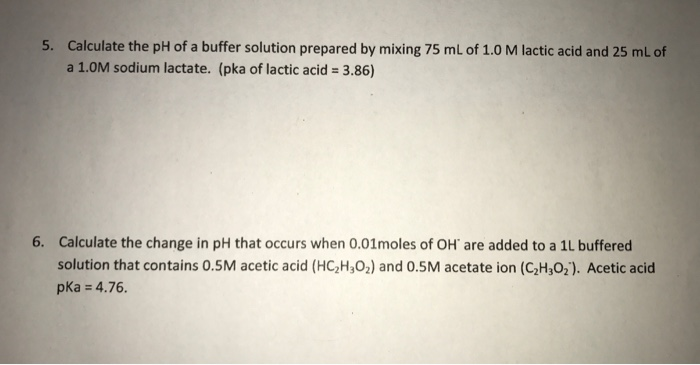Calculate the pH of a buffer solution prepared by mixing 75 mL of 1.0 M lactic acid and 25 mL of a 1.0M sodium lactate. (pka of lactic acid = 3.86) Calculate the change in pH that occurs when 0.01moles of OH are added to a 1L buffered solution that contains 0.5M acetic acid (HC_2H_3O_2) and 0.5M acetate ion (C_2H_3O_2). Acetic acid pka = 4.76.

• ### Calculate the thoeretical pH of Solution B (10mL of 0.2M acetic acid and 10 mL of...

Calculate the thoeretical pH of Solution B (10mL of 0.2M acetic acid and 10 mL of 0.1M sodium hydroxide). Then calculate the theoretical pH after an addition of 1 mL of 0.0992M of HCl. Initial pH: 4.66, pH after addition of acid: 4.66 Calculate the theoretical pH of solution B (10 mL of 0.2M sodium acetate and 10 mL of 0.1M HCl). Then calculate the theoretical pH after an additon of 1 mL of 0.997M of NaOH. Initial pH: 4.81,...

• ### QUESTION 28 What is the pH of a solution containing 0.1 M acetic acid (pka =...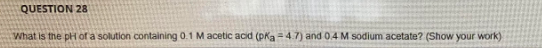QUESTION 28 What is the pH of a solution containing 0.1 M acetic acid (pka = 4.7) and 0.4 M sodium acetate? (Show your work)

• ### A. What is the pH of 2L of a buffer solution containing 80.0 grams of lactic...

A. What is the pH of 2L of a buffer solution containing 80.0 grams of lactic acid (CH3CH(OH)COOH) (90.08 g/mol) and 100.0 grams of sodium lactate (CH3CH(OH)COONa) (112.06 g/mol)? B. What will be the pH of the solution formed from adding 20mL of 8.0 M HCl to the buffer described in Part A of this question? (Ignore the change in volume) For part A, because we aren't given pKa, am I right to assume that we'd be finding the molarity...

• ### 1. Calculate the pKa of lactic acid, given that when the concentration of lactic acid is...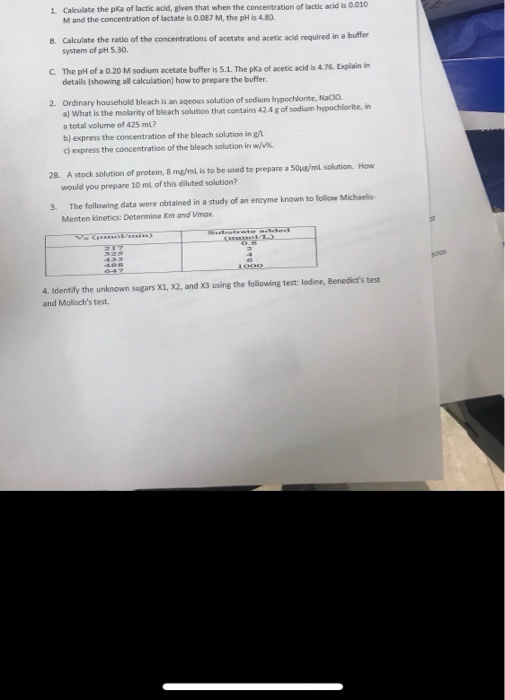1. Calculate the pKa of lactic acid, given that when the concentration of lactic acid is 0.010 M and the concentration of lactate is 0.087 M, the pH is 4.80 B. Calculate the ratio of the concentrations of acetate and acetic acid required in a buffer system of pH 5.30 C The pH of a 0.20 M sodium acetate buffer is 5.1. The pKa of acetic acid is 4.76. Explain in details (showing all calculation) how to prepare the buffer...

• ### 2.) Consider a 1.00L of buffer that is 0.139M in lactic acid (C3H5O3H) and 0.134M in sodium lactate (C3H5O3- Na+). Calcu...

2.) Consider a 1.00L of buffer that is 0.139M in lactic acid (C3H5O3H) and 0.134M in sodium lactate (C3H5O3- Na+). Calculate the pH of the buffer after adding 0.0500 mol of HCl into the buffer solution. (Assume no volume change) pKa of lactic acid is 3.86